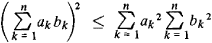# Cauchy's Inequality

The following article is from The Great Soviet Encyclopedia (1979). It might be outdated or ideologically biased.

## Cauchy’s Inequality

an inequality for finite sums having the formIt is one of the most important and commonly used inequalities and was proved by A. Cauchy (1821). The integral analog of Cauchy's inequality was established by the Russian mathematician V. la. Buniakovskii, while an important generalization of Cauchy's inequality was made by the German mathematician L. O. Hölder.

Mentioned in ?
References in periodicals archive ?
If [([z.sub.k]).sub.k [greater than or equal to] 0] is a basis for E and if Cauchy's inequality (8) is satisfied, then [Z.sub.n] is a continuous linear operator on E, orthonormal to [([z.sub.k]).sub.k [greater than or equal to] 0].
In this case, Cauchy's inequality (8) takes the form (see )
The basis for H(r) is taken, as before, to be the Appell sequence [([P.sub.n](x)).sub.n [greater than or equal to] 0] which accords to Cauchy's inequality and it is also an absolute basis.
From (3.30), (3.36) and the Cauchy's inequality we get
From (3.65), (3.70) and the Cauchy's inequality we get
c) For p = q = 2 in inequality (10), we obtain a refinement of Cauchy's inequality
and after using Cauchy's inequality, the estimate 1/k+n < 1/n, and the definition of the moments in (1.5),
To estimate [U.sup.1,1.sub.n], we use Cauchy's inequality
With Lemma 1 and Cauchy's inequality, we get (for t [greater than or equal to] 2)

Site: Follow: Share:
Open / Close